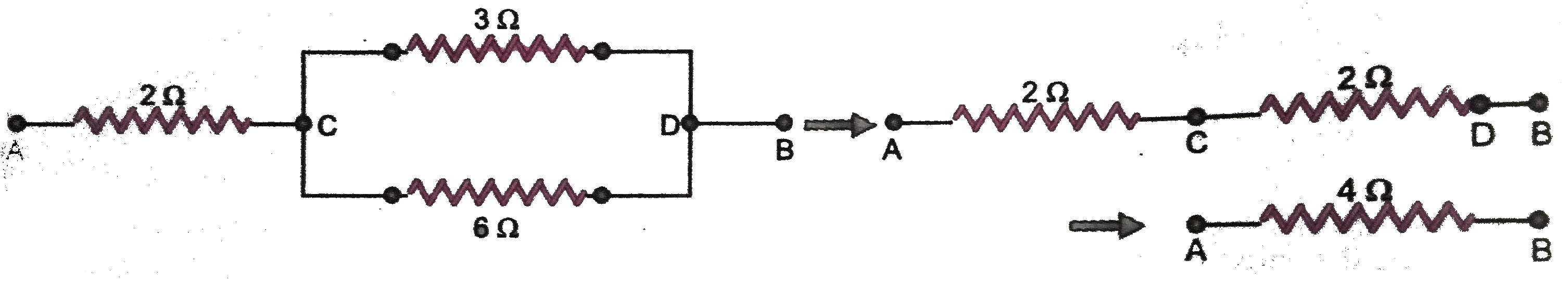# How can three resistors of resistances 2 Omega,3 Omega, and 6 Omega be connected to give a total resistance of (a) 4 Omega (b) 1 Omega ?

51 views
in Physics
closed
How can three resistors of resistances 2 Omega,3 Omega, and 6 Omega be connected to give a total resistance of
(a) 4 Omega
(b) 1 Omega ?

by (71.9k points)
selected by

(a) To get a total resistance of 4 Omega from resistors of resistances 2 Omega,3 Omega and 6 Omega , the resistors of resistances 3 Omega and 6 Omega should be connected in parallel. Their equivalent resistance is ((3 xx 6)/(3 + 6) Omega = 2 Omega).
This combination of equivalent resistance 2 Omega should be connected in series with the resistor of resistance 2 Omega so that equivalent resistance of three resistors becomes 2 Omega + 2 Omega = 4 Omega as shown in (Fig. 3.34).
(b) To get a resistance of 1 Omega from three given resistors of resistances 2 Omega,3 Omega,6 Omega, we should connect all of them in parallel. The equivalent resistance is given by
(1)/(R_p) = (1)/(2)+(1)/(3)+(1)/(6)= (3 + 2 + 1)/(6) = (6)/(6) = 1 i.e., R_p = 1 Omega..